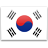S Q L T

S Q L T

S L Q T

S L Q T

S L Q T

L Q S T

L Q S T

L Q S T

L Q T S

L Q T S

L Q T S

L Q T S

L Q T S

Q L T S

L S Q T

L Q T S

L Q T S

L Q T S

L Q T S

L Q S

L T Q S

Q T L